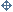<58r>

Sir

In the Scholium you write of, the words vel Hyperbolæ in the 3d line are to be struck out, & in the 5t or 6t line the words quæ sit ad $\underset{_}{GK}$ should be quæ sit ad $\frac{1}{2}GK$. I send you inclosed the beginning of this Scholium with the 63d figure as I would have them printed. I thank you heartily for giving me notice that it was amiss. The ground of the transmutation of a trapezium into a parallelogram I lay down pag 87 in these wo{rds:} "Nam rectæ quævis convergentes transmutantur in parallelas, adhibendo pro radio ordinato primo AO lineam quamvis rectam quæ per concursum convergentium transit: id adeo quia concursus ille hoc pacto abit in infinitum, lineæ autem parallelæ sunt quæ ad punctum infinitè distans tendunt." In the figure pag 86 conceive the curve HGI to be produced both ways till it meet & intersect it self any where in the radius ordinatus primus AO: & when the point G moving up & down in the curve HI arrives at that intersection point, I say the point g moving in like manner up & down in the curve hi will become infinitely distant. For the point G falling upon the line OA, the point D will fall upon the point A & the line OD upon the line OA & so becoming parallel to AB their intersection point d will become infinitely distant & consequently the line dg will become infinitely distant & so will its point g. Q.E.D. So then if any two lines of the primary figure HGID intersect in the radius ordinatus primus AO their intersection in the new figure hgid shall become infinitely distant & therefore if the two intersecting lines be right ones they shall become parallel. For right lines which tend to a point infinitely distant do not intersect one another & diverge but are par{allel.} Therefore if in the primary figure there be any Trapezium wh{ose} opposite sides converge to points in the radius ordinatus primus {OA} those sides in the new figure shal become parallel & so the trapezium be converted into a parallelogram.

The printed sheets I intend to looke over. Mr Paget in his stay here has noted these errata of which the 3d is a fault in the Copy.

p. 6, l. 27. velocitate. p. 8{,} l. 19. –tur. Sunt. p. 14{,} l. 30: reciproce ut $\underset{_}{DO}$. p. 18{,} l. 1 recta.       I wish the printer be carefull to mend all you note. Sir I am very sensible of the great trouble you are at in this business, & the great care you take about it. Pray take your own time. And if you meet with any thing else which you think need either correcting or further explaining be pleased to signify it to

Your humble & Obliged Servant

Is. Newton.

Trin. Coll.
Octob. 18. 1686.

My thanks for your note of
{De la Hire}

<58v> < insertion from the left margin of f 58v >

Mr Newton
Octob. 18. 1686

< text from f 58v resumes > <58ar> < insertion from f 58r >

Errata sensum turbantia sic Emenda.

pag. 14 lin. 30 leg. . pag. 18 l. 1 recta. p. 61 l. 22 et p. 62 lin. 2 pro $\underset{_}{AC}$ lege $\underset{_}{AB}$.p. 95. l. 3 post majori adde & perpendicularia minori. pag. 96 l. 30 & 31 lege $\underset{_}{ABCdef}$, et l. 32. abcDEF p. 104 l. 16 pro ${GO}^{q}+{\stackrel{_}{HG-PO}}^{q})$ lege ${HP}^{q}={GO}^{q}+{\stackrel{_}{PO-HG}}^{q})$ pag. 105 lin 7 pro G scribe H. p. 118 l. 17 pro CP lege PfB et lin 19 pro CP lege BP. p. 122 lin 28 pro L scribe M. p. 125. l. 16. pro omnibus altitudinibus lege omnibus æqualibus altitudinibusp 209. l. 18. pro $SL×{SI}^{\frac{1}{2}}$ lege $SL×{SI}^{\frac{3}{2}}$. pag 2 lin. 11 pro lege $\underset{_}{2{B}^{3}}$ et dele reciproce{.}

{p.} 416 l. 17 pro $\frac{1}{32000}$ lege $\frac{1}{3200}${.} p. 494 L. 4 pro lege .

< text from f 58ar resumes >

© 2019 The Newton Project

Professor Rob Iliffe
Director, AHRC Newton Papers Project

Scott Mandelbrote,
Fellow & Perne librarian, Peterhouse, Cambridge

Faculty of History, George Street, Oxford, OX1 2RL - newtonproject@history.ox.ac.uk

Privacy Statement